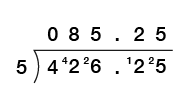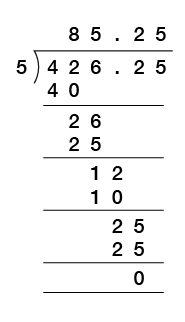Science, Maths & Technology

### Become an OU studentEveryday maths 1 (Wales)

Start this free course now. Just create an account and sign in. Enrol and complete the course for a free statement of participation or digital badge if available.

# Division

## Dividing decimals by 10, 100 and 1 000

When you divide a decimal number by 10, all the numbers get 10 times smaller, so the decimal point moves one place to the left.

When you divide by 100, all the numbers get 100 times smaller, so the decimal point moves two places to the left.

When you divide by 1 000, all the numbers get 1 000 times smaller, so the decimal point moves three places to the left.

Watch the following clip which will show you the correct method for dividing decimal numbers by 10, 100 or 1 000.

Interactive feature not available in single page view (see it in standard view).

Now try the following activity.

### Activity 30: Dividing decimals by 10, 100, 1 000

Calculate the following:

1. 57.08 ÷ 10
2. 6.09 ÷ 10
3. 433.57 ÷ 100
4. 51.2 ÷ 100
5. 899.34 ÷ 1 000
6. 67.51 ÷ 1 000

1. 5.708
2. 0.609
3. 4.3357
4. 0.512
5. 0.89934
6. 0.06751

## Dividing a decimal number by a whole number

When you divide a decimal number by a whole number, you divide as normal and keep the decimal point in line.

The following video includes some examples:

Interactive feature not available in single page view (see it in standard view).

Now try the following activity.

### Activity 31: Dividing a decimal by a whole number

Calculate the following:

1. 8.46 ÷ 6
2. 79.9 ÷ 5
3. 70.38 ÷ 9
4. 423.06 ÷ 3
5. 0.845 ÷ 5
6. 301.14 ÷ 8
7. If an electricity bill costs £527.40 per year, how much does it cost per month?
8. A taxi bill costs £34.80. If this is shared by four friends, how much will each person pay?

1. 1.41
2. 15.98
3. 7.82
4. 141.02
5. 0.169
6. 37.6425
7. £43.95 per month
8. £8.70 each

## Dividing a decimal number by another decimal number

When you divide a decimal number by another decimal number, you first have to change the number you are dividing by into a whole number. You do this by multiplying by either 10, 100 or 1 000.

### Example: Dividing a decimal number by another decimal number

Calculate the following:

4.2625 ÷ 0.05

#### Method

The number you are dividing by is 0.05, so to make it a whole number you multiply by 100 (that is, move the decimal point two places to the right):

0.05 × 100 = 5

You then multiply the number you are dividing into by the same amount, in this example 100. The number you are dividing into is 4.2625, so:

4.2625 × 100 = 426.25

Note how you do not have to change the number that you are dividing into a whole number.

The calculation you now have is:

426.25 ÷ 5

Using the short division method, the calculation would be:This could also be done using the long division method if this is the method you prefer to use:However you do the division, just make sure the decimal point goes in line in the answer with where it is in the number you are dividing.

Now try the following activity.

### Activity 32: Dividing a decimal by a decimal

Calculate the following, showing your answers to 2 dp when not exact:

1. 1.5 ÷ 0.5
2. 10.8 ÷ 0.03
3. 13.25 ÷ 0.5
4. 2.5 ÷ 0.04
5. 56.9 ÷ 3.1
6. 5.75 ÷ 1.1
7. If a tea urn holds 12.5 litres, how many 0.2 litre cups of tea will it provide?
8. A garden path is 9.5 metres long and paving slabs are 0.25 metres long each. How many paving slabs will it take to cover the length of the path?